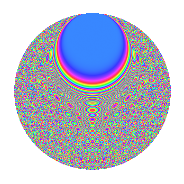# Properties

 Label 2015.2.djLevel 2015 Weight 2 Character orbit dj Rep. character $$\chi_{2015}(347,\cdot)$$ Character field $$\Q(\zeta_{12})$$ Dimension 880 Sturm bound 448

# Related objects

## Defining parameters

 Level: $$N$$ = $$2015 = 5 \cdot 13 \cdot 31$$ Weight: $$k$$ = $$2$$ Character orbit: $$[\chi]$$ = 2015.dj (of order $$12$$ and degree $$4$$) Character conductor: $$\operatorname{cond}(\chi)$$ = $$2015$$ Character field: $$\Q(\zeta_{12})$$ Sturm bound: $$448$$

## Dimensions

The following table gives the dimensions of various subspaces of $$M_{2}(2015, [\chi])$$.

Total New Old
Modular forms 912 912 0
Cusp forms 880 880 0
Eisenstein series 32 32 0

## Trace form

 $$880q - 4q^{2} - 4q^{5} - 12q^{6} + 2q^{7} - 32q^{8} + O(q^{10})$$ $$880q - 4q^{2} - 4q^{5} - 12q^{6} + 2q^{7} - 32q^{8} + 2q^{10} - 12q^{11} - 18q^{12} - 6q^{13} + 416q^{16} - 6q^{17} + 4q^{18} - 68q^{20} + 12q^{23} - 4q^{25} - 12q^{26} - 28q^{28} - 30q^{30} + 16q^{31} + 18q^{32} + 20q^{33} - 10q^{35} + 436q^{36} - 32q^{38} - 2q^{40} - 4q^{41} - 54q^{42} + 6q^{43} + 42q^{45} + 24q^{46} - 8q^{47} - 6q^{48} - 20q^{50} - 16q^{51} - 30q^{52} - 12q^{53} + 4q^{56} - 12q^{57} - 48q^{61} + 76q^{62} + 32q^{63} - 24q^{65} - 32q^{66} - 6q^{67} - 224q^{70} + 120q^{71} - 36q^{72} - 12q^{73} - 72q^{75} - 136q^{76} + 70q^{78} - 8q^{80} - 760q^{81} + 12q^{82} - 114q^{83} + 48q^{85} + 24q^{86} + 34q^{87} - 30q^{88} + 8q^{90} - 24q^{91} - 40q^{93} + 18q^{95} - 40q^{97} - 76q^{98} + O(q^{100})$$

## Decomposition of $$S_{2}^{\mathrm{new}}(2015, [\chi])$$ into newform subspaces

The newforms in this space have not yet been added to the LMFDB.

## Hecke Characteristic Polynomials

There are no characteristic polynomials of Hecke operators in the database